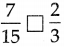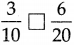Balbharti Maharashtra Board Class 5 Maths Solutions Chapter 5 Fractions Problem Set 19 Textbook Exercise Important Questions and Answers.

## Maharashtra State Board Class 5 Maths Solutions Chapter 5 Fractions Problem Set 19

Write the proper symbol from < , > , or = in the box.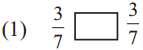=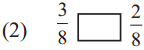>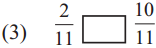<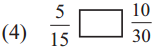=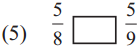>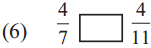>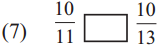>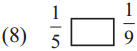>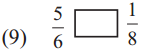>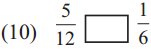>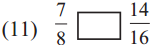=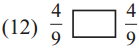=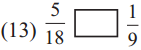>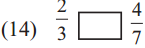>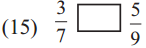<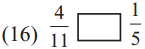>

Example (1) 3/7 + 2/7 = ?
Let us divide a strip into 7 equal parts. We shall colour 3 parts with one colour and 2 parts with another.
The part with one colour is 3/7, and that with the other colour is 2/7.
The total coloured part is shown by the fraction 5/7.
It means that, $$\frac{3}{7}+\frac{2}{7}=\frac{3+2}{7}=\frac{5}{7}$$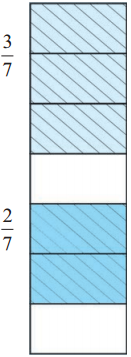Example (2) Add : $$\frac{3}{8}+\frac{2}{8}+\frac{1}{8}$$
The total coloured part is $$\frac{3}{8}+\frac{2}{8}+\frac{1}{8}=\frac{3+2+1}{8}=\frac{6}{8}$$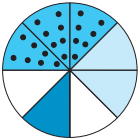When adding like fractions, we add the numerators of the two fractions and write the denominator as it is.
Example (3) Add : 2/6 + 4/6 $$\frac{2}{6}+\frac{4}{6}=\frac{2+4}{6}=\frac{6}{6}$$
However, we know that 6/6 means that all 6 of the 6 equal parts are taken. That is, 1 whole figure is taken. Therefore, 6/6 = 1.

Note that:
If the numerator and denominator of a fraction are equal, the fraction is equal to one.
That is why, $$\frac{7}{7}=1 ; \frac{10}{10}=1 ; \frac{2}{5}+\frac{3}{5}=\frac{2+3}{5}=\frac{5}{5}=1$$
Remember that, if we do not divide a figure into parts, but keep it whole, it can also be written as 1.
This tells us that $$1=\frac{1}{1}=\frac{2}{2}=\frac{3}{3}$$ and so on.
You also know that if the numerator and denominator of a fraction have a common divisor, then the fraction obtained by dividing them by that divisor is equivalent to the given fraction.
$$\frac{5}{5}=\frac{5 \div 5}{5 \div 5}=\frac{1}{1}=1$$